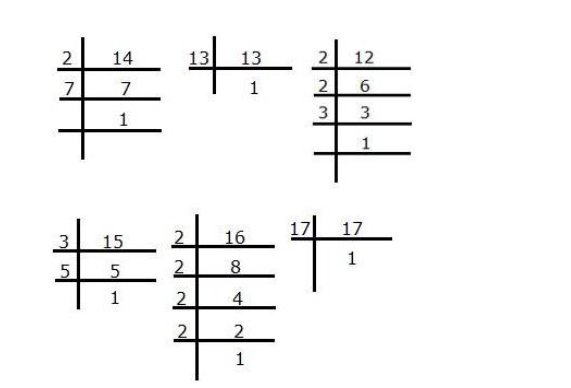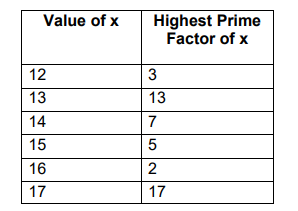# Let X = {12, 13, 14, 15, 16, 17} and f

Question:

Let $X=\{12,13,14,15,16,17\}$ and $f: A \rightarrow Z: f(x)=$ highest prime factor of $x$.

Find range (f)

Solution:

Given: f(x) = highest prime factor of x

And since $x \in A, A=\{12,13,14,15,16,17\}$

Value of $x$ can only be $12,13,14,15,16,17$

Doing prime factorization of the above, we getHence, range of f = {2, 3, 5, 7, 13, 17}